“Very easy to understand without oversimplifying some of the more advanced concepts.”

Jordan Boulger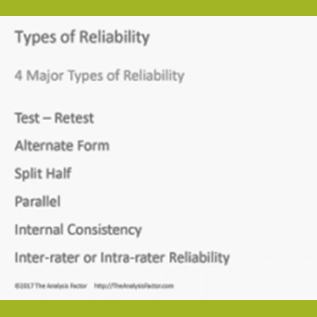Reliability is the key to achieving soundness in measurements that have potential for error.  Not only will you learn about why reliability matters, but also the different types and how to apply them.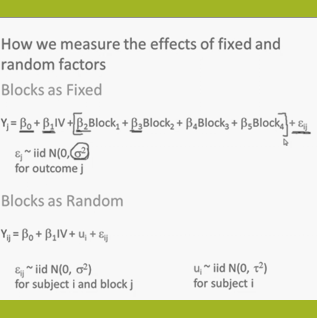What is the difference between fixed and random factors in Mixed Models? Learn the criteria to decide which one you have.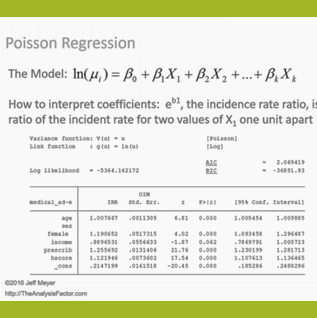Learn when you need to use Poisson or Negative Binomial Regression in your analysis, how to interpret the results, and how they differ from similar models.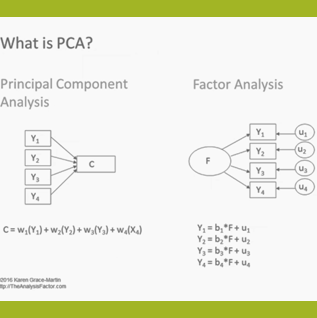Summarize common variation in many variables... into just a few. Learn the 5 steps to conduct a Principal Component Analysis and the ways it differs from Factor Analysis.

"Listening to your webinars is a much easier way of brushing up than trying to reread through my grad school books.”

Kara Snoke, Biostatistician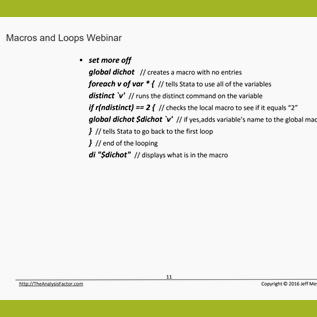Learn to run lengthy, repetitive tasks in Stata quickly and easily by setting up these two useful Stata tools in a do-file.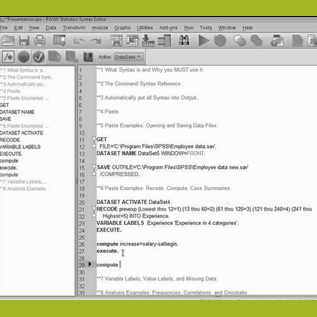Do you want to know how to use SPSS syntax for data manipulation and analyses? You will witness all the advantages that come with using syntax, especially one that is already built-in.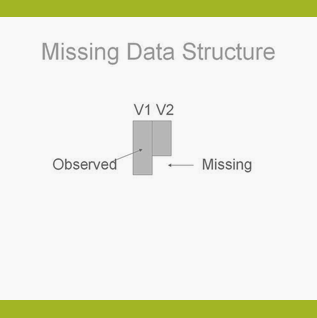Learn the different types of missing data and how they affect which approach to take in any situation.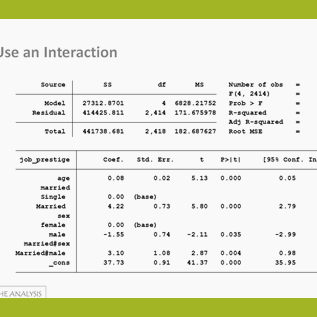While you’re worrying about which predictors to enter, you might be missing issues that have a big impact your analysis. This training will help you achieve more accurate results and a less-frustrating model building experience.

“The webinars are a great way to learn or refresh on a particular topic area.”

Frances Aranda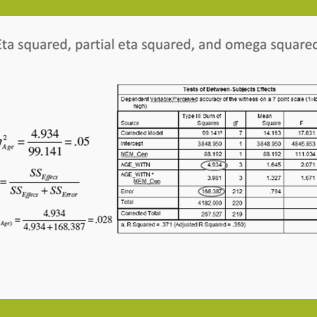Statistical software doesn't always give us the effect sizes we need. Learn some of the common effect size statistics and the ways to calculate them yourself.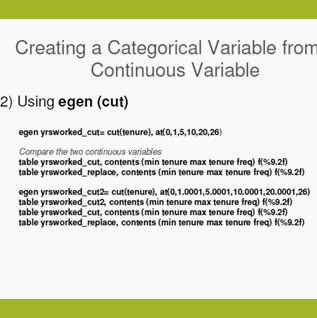This is a one-hour demonstration for new and intermediate Stata users on how to simplify your next analysis in Stata.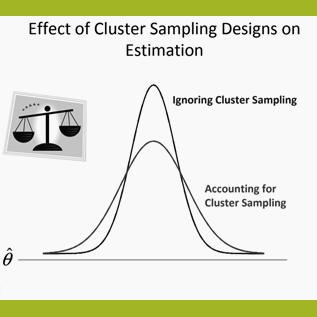Are you analyzing data from a nationally representative survey? Learn about the different types of sampling techniques and their effects on data analysis.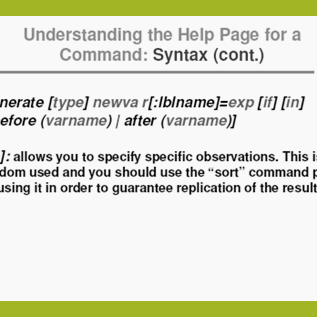Do you want to reduce the amount of time you spend figuring how to get Stata to do what you need? Join us as we explore different commands and resources to help you create accurate analyses in significantly less time.
“Thank you so much. You are one heck of a teacher!”

Dan Lofald, Teacher Educator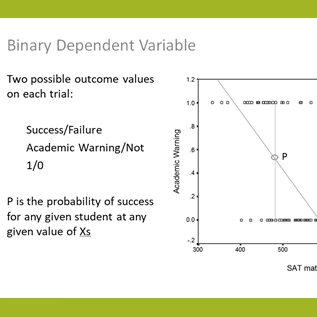Get beyond the frustration of learning odds ratios, logit link functions, and proportional odds assumptions on your own. See the incredible usefulness of logistic regression and categorical data analysis in this one-hour training.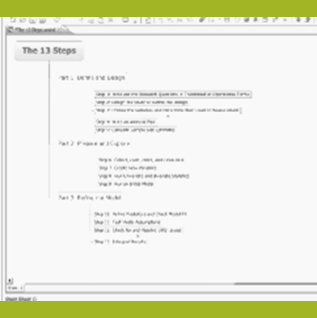Learn the efficient and straightforward way to do any statistical modeling –  ANOVA, Linear Regression, Poisson Regression, Multilevel Model, or whatever else your project requires.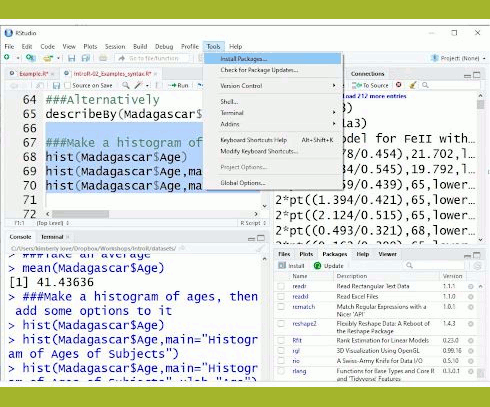This is an introduction to the use of R statistical software for data manipulation, calculation, and graphical display.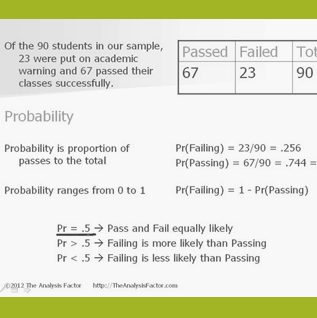Despite the way the terms are used in common English, odds and probability are not interchangeable. Join us to see how they differ, what each one means, and how to tame that tricky beast: Odds Ratios.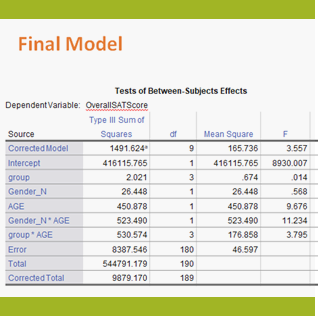Learn the approach for understanding coefficients in that regression as we walk through output of a model that includes numerical and categorical predictors and an interaction.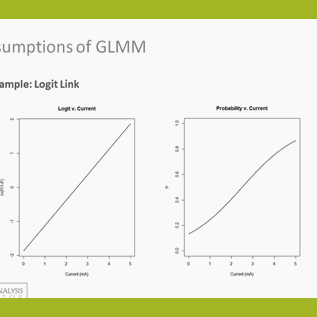Ready to take your statistics proficiency to the next level? Learn all about Generalized Linear Mixed Models and when and how to apply it to your data analysis.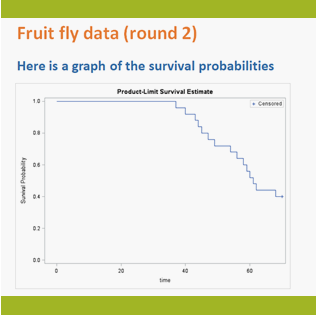Learn the key tools necessary to learn Survival Analysis in this brief introduction to censoring, graphing, and tests used in analyzing time-to-event data.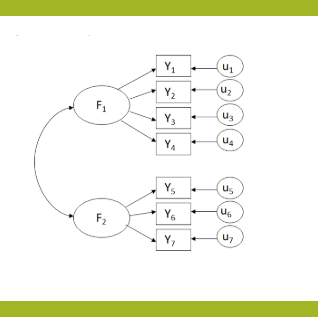Confirmatory factor analysis (CFA) and path models make up two core building blocks of SEM. Learn how these help you understand how SEM is used.
Sign up to our mailing list to receive updates about new webinars:
[bot_catcher]